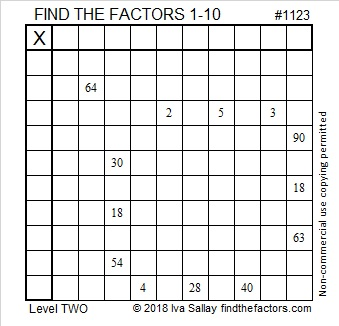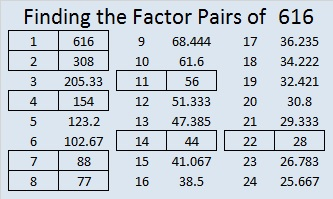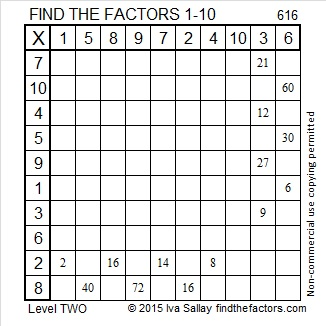# 1123 and Level 2

All the clues in one of the rows of this Level 2 puzzle are prime numbers. The only common factor they have is 1. That fact will get you started with this puzzle which I am sure you can complete if you just give it a try!Print the puzzles or type the solution in this excel file: 10-factors-1121-1133

1123 is also a prime number. Here are some facts about it.

• 1123 is a prime number.
• Prime factorization: 1123 is prime.
• The exponent of prime number 1123 is 1. Adding 1 to that exponent we get (1 + 1) = 2. Therefore 1123 has exactly 2 factors.
• Factors of 1123: 1, 1123
• Factor pairs: 1123 = 1 × 1123
• 1123 has no square factors that allow its square root to be simplified. √1123 ≈ 33.51119

How do we know that 1123 is a prime number? If 1123 were not a prime number, then it would be divisible by at least one prime number less than or equal to √1123 ≈ 33.5. Since 1123 cannot be divided evenly by 2, 3, 5, 7, 11, 13, 17, 19, 23, 29 or 31, we know that 1123 is a prime number.Stetson.edu reminds us that 1, 1, 2, 3 are the first four numbers in the Fibonacci sequence.

1123 is the sum of five consecutive prime numbers:
211 + 223 + 227 + 229 + 233 = 1123

1123 is palindrome 797 in BASE 12 because 7(12²) + 9(12) + 7(1) = 1123, and
it’s repdigit 111 in BASE 33 because 33² + 33 + 1 = 1123

# 616 and Level 2

The Padovan sequence produces a lovely spiral of equilateral triangles similar to the spiral made from golden rectangles and the Fibonacci sequence.

The Padovan sequence begins with the following numbers: 1, 1, 1, 2, 2, 3, 4, 5, 7, 9, 12, 16, 21, 28, 37, 49, 65, 86, 114, 151, 200, 265, 351, 465, 616, 816, 1081 …

The first two numbers in the Fibonacci sequence are both 1’s. After that a number, n, in the Fibonacci sequence is found by adding together (n-2) and (n-1).

The first three numbers in the Padovan sequence are all 1’s. After that a number, n, in the Padovan sequence is found by adding together (n-3) and (n-2), and 616 is one of those numbers.Print the puzzles or type the solution on this excel file: 10 Factors 2015-09-14

—————————————————————————————————

• 616 is a composite number.
• Prime factorization: 616 = 2 x 2 x 2 x 7 x 11, which can be written 616 = (2^3) x 7 x 11
• The exponents in the prime factorization are 1, 3, and 1. Adding one to each and multiplying we get (3 + 1)(1 + 1)(1 + 1) = 4 x 2 x 2 = 16. Therefore 616 has exactly 16 factors.
• Factors of 616: 1, 2, 4, 7, 8, 11, 14, 22, 28, 44, 56, 77, 88, 154, 308, 616
• Factor pairs: 616 = 1 x 616, 2 x 308, 4 x 154, 7 x 88, 8 x 77, 11 x 56, 14 x 44, or 22 x 28
• Taking the factor pair with the largest square number factor, we get √616 = (√4)(√154) = 2√154 ≈ 24.819347.—————————————————————————————————